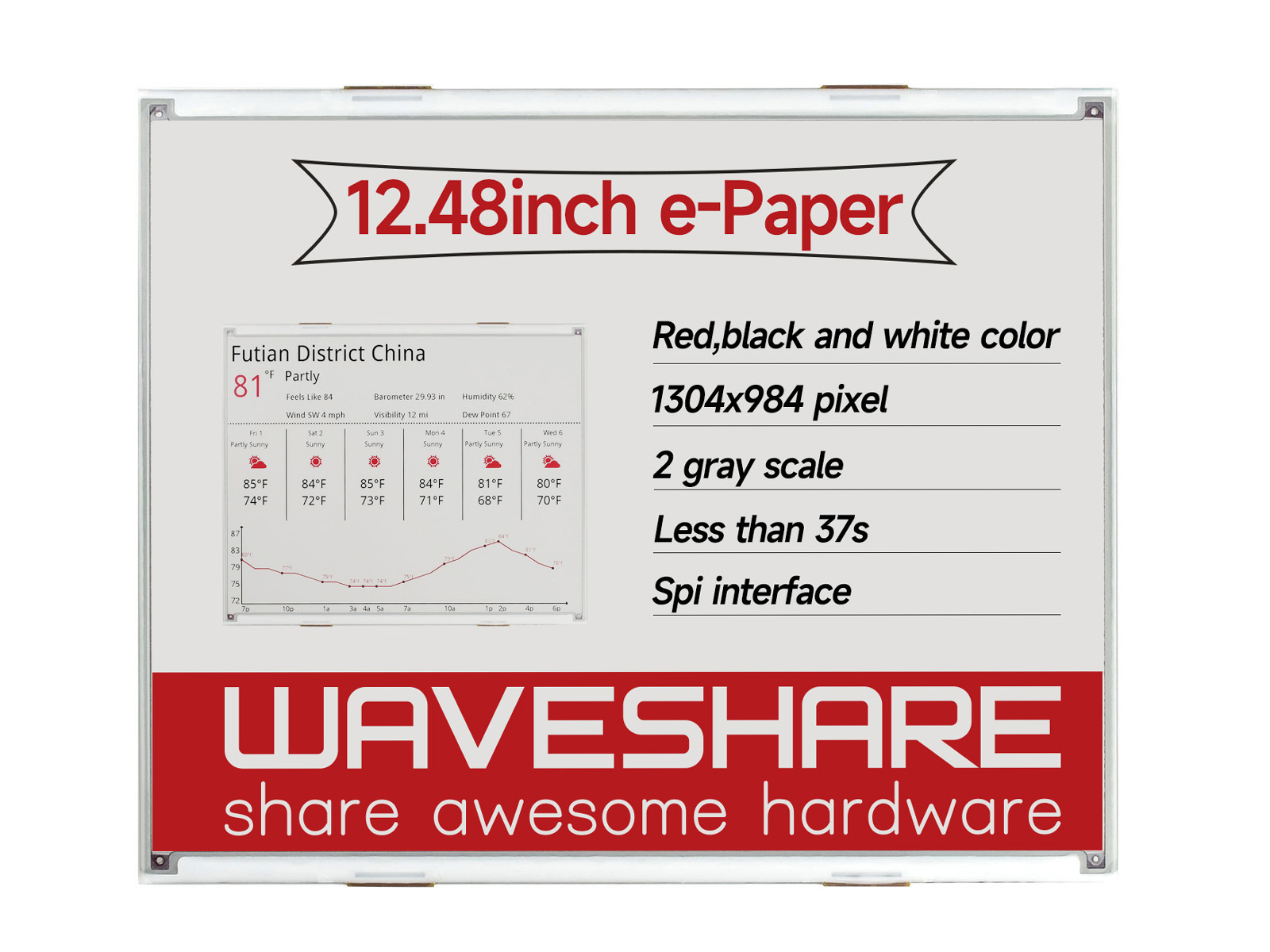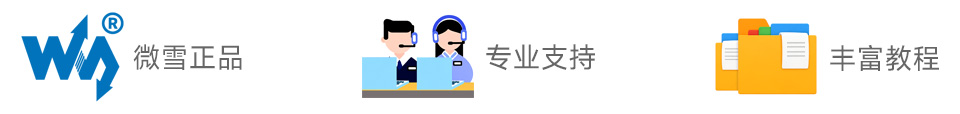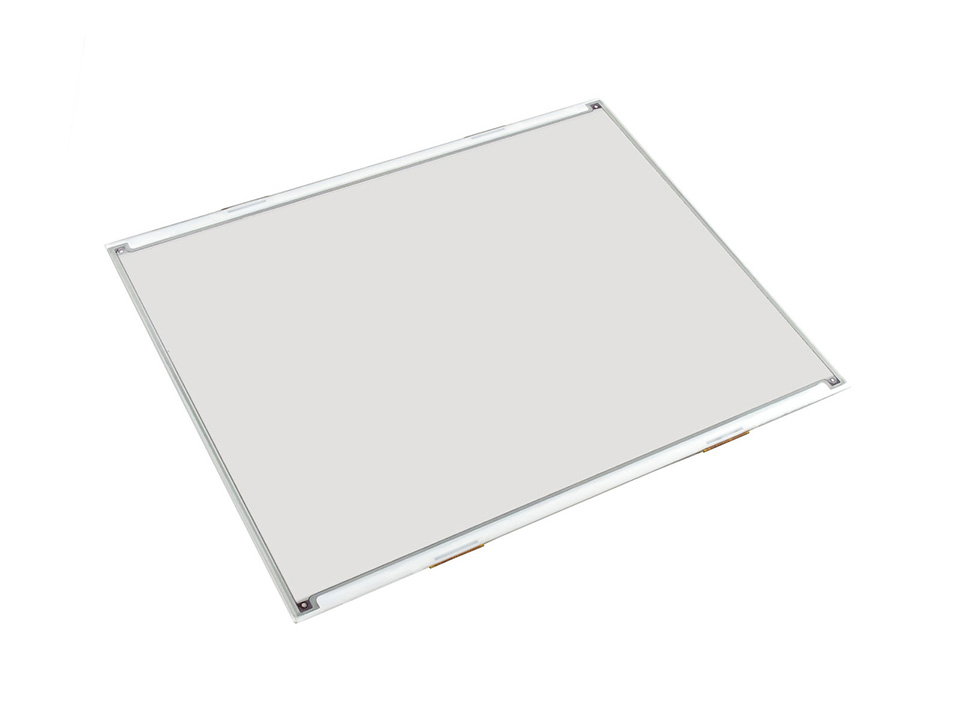# 12.48寸电子墨水屏裸屏 1304×984分辨率 红黑白三色

### 缺货

12.48inch e-Paper (B)
SKU
17292

Waveshare
• 产品详情
• 产品资料
• 配置清单

# 12.48英寸电子墨水屏祼屏

• 尺寸 12.48英寸
• 分辨率 1304 × 984
• 可视角度 >170°
• 显示颜色 红、黑、白
• 灰度级别 2
• 通信接口 SPI
• 显示面板 电子墨水屏
• 显示体验 接近纸质
• 显示环境 环境光下可见
• 显示方式 反射式，不主动发光

## 我的特点

• 无需背光，断电后可长时间保持最后一屏的显示内容
• 功耗非常低，基本只在刷新时耗电
• 提供完善的配套资料手册(Raspberry Pi/Arduino/STM32/ESP32等示例程序)

## 我的参数

工作电压 显示颜色 3.3V 红、黑、白 3-wire SPI、4-wire SPI 2 261.5 × 211.0 × 1.18mm 16s 252.976 × 190.896mm 82.5mW(typ.) 0.194 × 0.194mm <0.01uA(接近0) 1304 × 984pixels >170°

## 产品选型

1.02inch e-Paper 黑、白 2 128×80 21.76 × 14.00 32.57 × 18.60 × 0.98 2 × SPI
1.54inch e-Paper 黑、白 2 200×200 27.00 × 27.00 37.32 × 31.80 × 1.05 2 × SPI
1.54inch e-Paper (B) 红、黑、白 2 200×200 27.60 × 27.60 37.32 × 31.80 × 1.05 8 × × SPI
2.13inch e-Paper 黑、白 2 250×122 48.55 × 23.70 59.20 × 29.20 × 1.05 2 × SPI
2.13inch e-Paper (B) 红、黑、白 2 212×104 48.55 × 23.70 59.20 × 29.20 × 0.98 15 × × SPI
2.13inch e-Paper (D) 黑、白 2 212×104 48.55 × 23.70 59.20 × 29.20 × 0.30 2 SPI
2.66inch e-Paper 黑、白 2 296×152 30.704 × 60.088 36.304 × 71.820 × 1.00 6 × SPI
2.66inch e-Paper (B) 红、黑、白 2 296×152 30.704 × 60.088 36.304 × 71.820 × 1.00 15 × × SPI
2.7inch e-Paper 黑、白 4 264×176 57.288 × 38.192 70.42 × 45.80 × 0.98 6 × × SPI
2.7inch e-Paper (B) 红、黑、白 2 264×176 57.288 × 38.192 70.42 × 45.80 × 0.98 15 × × SPI
2.9inch e-Paper 黑、白 2 296×128 66.89 × 29.05 79.00 × 36.70 × 1.05 2 × SPI
2.9inch e-Paper (B) 红、黑、白 2 296×128 66.90 × 29.06 79.00 × 36.70 × 1.18 15 × × SPI
2.9inch e-Paper (D) 黑、白 2 296×128 66.90 × 29.06 79.00 × 36.70 × 0.34 2 SPI
3.7inch e-Paper 黑、白 4 480×280 47.32 × 81.12 54.90 × 93.30 × 0.78 3 × SPI
4.01inch e-Paper (F) 7彩色 2 640×400 86.40 × 54.00 96.80 × 68.70 × 0.91 15 × × SPI
4.2inch e-Paper 黑、白 4 400×300 84.80 × 63.60 91.00 × 77.00 × 1.18 4 × × SPI
4.2inch e-Paper (B) 红、黑、白 2 400×300 84.80 × 63.60 90.10 × 77.00 × 1.18 15 × × SPI
4.2inch e-Paper (C) 黄、黑、白 2 400×300 84.80 × 63.60 90.10 × 77.00 × 1.18 15 × × SPI
5.65inch e-Paper (F) 7彩色 2 600×448 114.9 × 85.8 125.4 × 99.5 × 0.91 <35 × × SPI
5.83inch e-Paper 黑、白 2 648×480 119.232 × 88.320 125.40 × 99.50 × 1.18 5 × × SPI
5.83inch e-Paper (B) 红、黑、白 2 648×480 119.232 × 88.320 125.40 × 99.50 × 1.18 20 × × SPI
5.83inch e-Paper (C) 黄、黑、白 2 600×448 118.80 × 88.26 125.40 × 99.50 × 1.18 26 × × SPI
6inch HD e-Paper 黑、白 16 1448×1072 122.356 × 90.60 138.40 × 101.80 × 1.01 <1 × 并口
7.5inch e-Paper 黑、白 2 800×480 163.20 × 97.92 170.20 × 111.20 × 1.18 5 × × SPI
7.5inch e-Paper (B) 红、黑、白 2 800×480 163.20 × 97.92 170.20 × 111.20 × 1.18 16 × × SPI
7.8inch e-Paper 黑、白 16 1872×1404 158.18 × 118.64 173.80 × 127.60 × 0.78 <1 × 并口
9.7inch e-Paper 黑、白 16 1200×825 202.80 × 139.43 218.80 × 156.425 × 1.15 <1 × 并口
10.3inch e-Paper 黑、白 16 1872×1404 209.66 × 157.25 216.70 × 174.40 × 0.78 <1 × 并口
10.3inch e-Paper (D) 黑、白 16 1872×1404 209.664 × 157.248 227.70 × 165.80 × 0.647 <1 并口
12.48inch e-Paper 黑、白 2 1304×984 252.976 × 190.896 261.50 × 211.00 × 1.18 <8 × × SPI
12.48inch e-Paper (B) 红、黑、白 2 1304×984 252.976 × 190.896 261.50 × 211.00 × 1.18 <16 × × SPI
13.3inch e-Paper 黑、白 16 1600×1200 270.40 × 202.80 285.80 × 213.65 × 0.78 <1 × 并口

## 资料及服务• 用户手册
• 驱动板原理图
• 示例程序
• 开发资料

（例程资料以链接中实际给出的为准）

## 配置清单

#### 12.48inch e-Paper (B) × 1